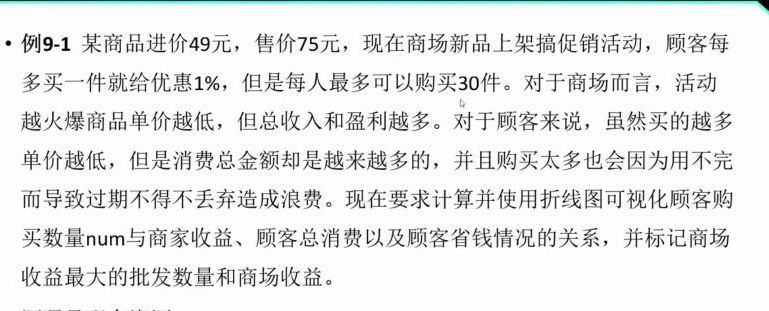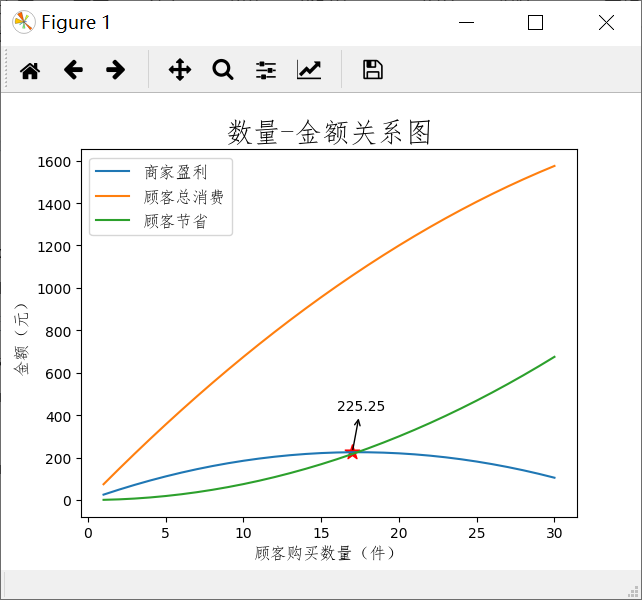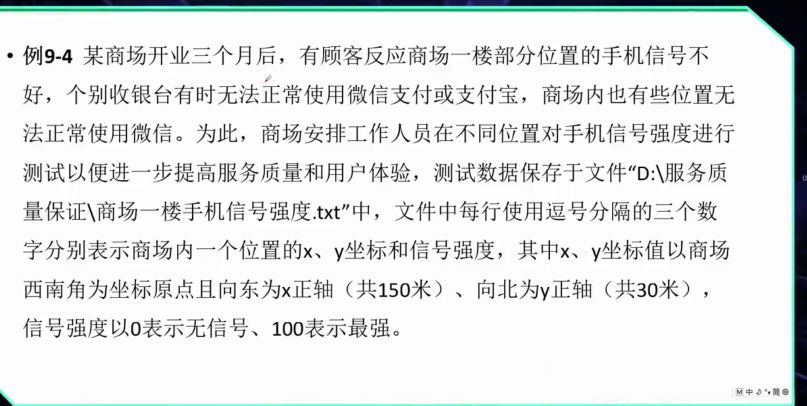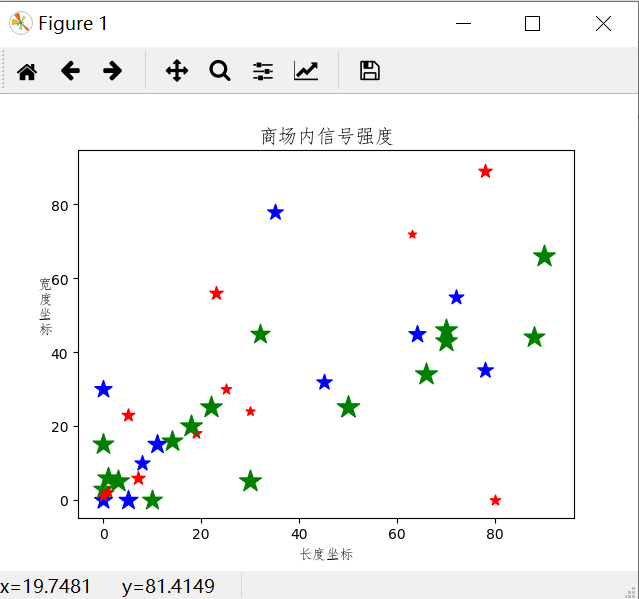# 字体调用问题：

1.导入数据库

``````from matplotlib.font_manager import FontProperties
``````

2.调用本机字体库

``````myfont=fm.FontProperties(fname=r'C:\Windows\Fonts\simfang.ttf',size=12)#通常在C:\Windows\Fonts下可以找到本机字体，选中其中一个字体，右键-属性，查看文件类型及字体名称，把这两个加到路径中即可，类似C:\Windows\Fonts\simfang.ttf，其中simfang是字体名，ttf是文件类型。
``````

3.之后每次使用字体可之间用myfont代替

``````plt.xlabel('顾客购买数量（件）',fontproperties=myfont)
``````

# 绘制折线图``````import matplotlib.pyplot as plt
import matplotlib.font_manager as fm #用来导入图例
from matplotlib.font_manager import FontProperties
myfont=fm.FontProperties(fname=r'C:\Windows\Fonts\simfang.ttf',size=12)
#进价与零售价
basePrice,salePrice=49,75
#计算购买num个商品时的单价，买的越多，单价越低
def compute(num):
return salePrice*(1-0.01*num)
numbers=list(range(1,31))#存储顾客购买数量
earns=[]#存储商场盈利情况
totalConsumption=[]#存储顾客消费总金额
saves=[]#存储顾客节省的总金额
#根据顾客购买数量计算三组数据
for num in numbers:
perPrice=compute(num)
earns.append(round(num*(perPrice-basePrice),2))
totalConsumption.append(round(num*perPrice,2))
saves.append(round(num*(salePrice-perPrice),2))#round函数是对数据进行四舍五入，2指保留两位小数
#绘制商家盈利和顾客节省的折线图，系统自动分配线条颜色
plt.plot(numbers,earns,label='商家盈利')
plt.plot(numbers,totalConsumption,label='顾客总消费')
plt.plot(numbers,saves,label='顾客节省')
#设置坐标轴标签

plt.xlabel('顾客购买数量（件）',fontproperties=myfont)#用fontproperties指定中文字体
plt.ylabel('金额（元）',fontproperties=myfont)
#设置图形标题
plt.title('数量-金额关系图',fontproperties=myfont,fontsize=20)#fontsize指定标题字号
#创建字体，设置图例

plt.legend(prop=myfont)#legend函数来设置图例
#计算并标记商家盈利最多的批发数量
maxEarn=max(earns)
bestNumbers=numbers[earns.index(maxEarn)]#index函数来找出maxEarn在earns中的位置
plt.scatter([bestNumbers],[maxEarn],marker='*',color='red',s=120)#用绘制散点图的方式进行标记
#使用annotate()函数在指定位置进行文本标注
plt.annotate(xy=(bestNumbers,maxEarn),#箭头终点坐标
xytext=(bestNumbers-1,maxEarn+200),#箭头起点坐标
s=str(maxEarn),#注释文本
arrowprops=dict(arrowstyle='<-'))#设置箭头形状
plt.show()#显示图像
``````# 绘制散点图``````
import matplotlib.pyplot as plt
import matplotlib.font_manager as fm
from matplotlib.font_manager import FontProperties
myfont=fm.FontProperties(fname=r'C:\Windows\Fonts\simfang.ttf',size=12)
xs=[]
ys=[]
strengths=[]
# 读取文件中的数据
with open(r'C:\Users\ht\PycharmProjects\图像绘制用的数据\散点图数据.txt') as fp:
for line in fp:
x,y,strength=map(int,line.split(','))
xs.append(x)
ys.append(y)
strengths.append(strength)#把文件中的数据分别添加到列表中
#绘制散点图
for x,y,s in zip(xs,ys,strengths):
if s<40:
color='r'
elif s<70:
color='b'
else:
color='g'
plt.scatter(x,y,s=s*3,c=color,marker='*')#这里的s表示散点符号的大小
plt.xlabel('长度坐标',fontproperties=myfont,fontsize=10)
plt.ylabel('宽\n度\n坐\n标',fontproperties=myfont,fontsize=10,
rotation='horizontal')#rotation用来设置文字方向
plt.title('商场内信号强度',fontproperties=myfont,fontsize=14)
plt.show()

``````### Git基础学习之Gitee的使用和设置SSH公钥_狂奔の蜗牛rz的博客-程序员宝宝_giteessh公钥怎么用

6.1 注册和使用gitee6.1.1 注册gitee账号1.什么是Gitee？Gitee又叫码云，但此“码云”非彼“马云”，并不是阿里的马云，而是一个基于Git的代码托管和研发协作平台官方网址：https://gitee.com/2.注册码云账号进入登录页面后，点击注册注册一个你的账号，使用手机号或者邮箱即可注册！这里我已经注册过了，所以就直接登录我的账号了！3.查看开源项目码云不仅是一个代码托管平台，同时也是一个开源项目交流社区，你可以在这里免费查看和下载别人的开源项目！查_1671465600

### linux 添加用户主目录,linux下修改用户主目录的方法_Zz 911的博客-程序员宝宝

linux下修改用户主目录的方法第一，修改/etc/passwd文件第二，usermod命令具体方法：第一种方法：vi /etc/passwd找到要修改的用户那几行，修改掉即可。此法很暴力，建议慎用。/etc/passwd文件格式登录名：加密口令：数字用户ID:数字组ID:注释字段：起始目录：shell程序用此方法修改了用户宿主目录后，会出现某些异常，如：bash环境异常，需要将用户原宿主目录下的...

### RDMA over Commodity Ethernet at Scale (II)_upupday19的博客-程序员宝宝

3.  DSCP-BASEDPFC在本小节中，我们测试了原始的基于VLAN的PFC面对的问题，并提出了基于DSCP的PFC方案。基于VLAN的PFC暂停帧中，VLAN TAG中包含了数据包优先级和VID，但是优先级和VID在部署中引发了两个严重的问题，因此提出了基于DSCP的PFC方案。暂停帧是一个二层帧，并没有VLAN标签，数据包的VLAN标签有四部分，TPID被固定为0x8100，DE